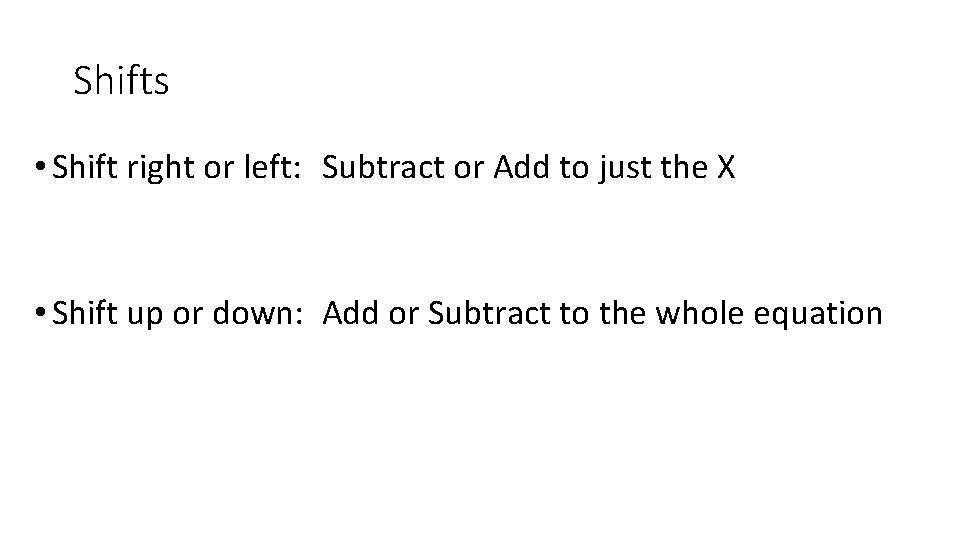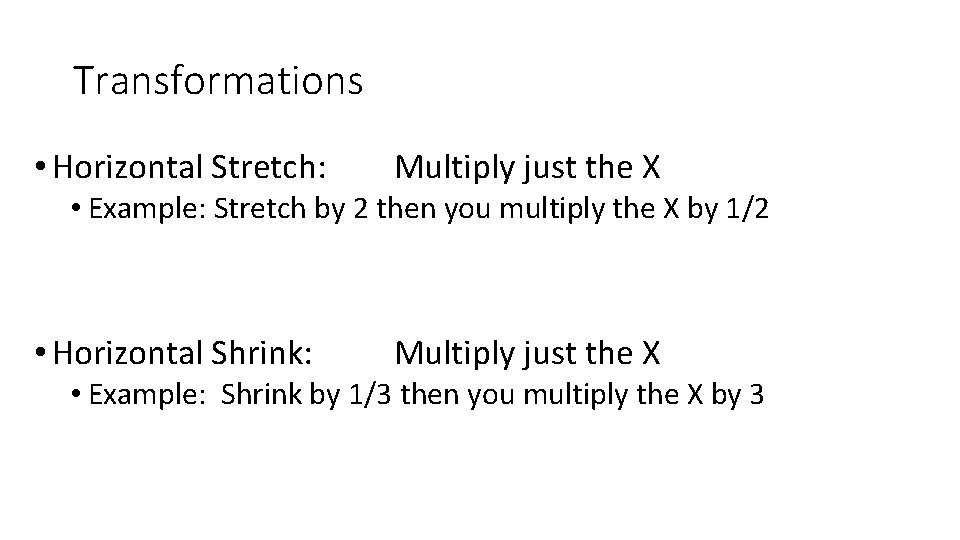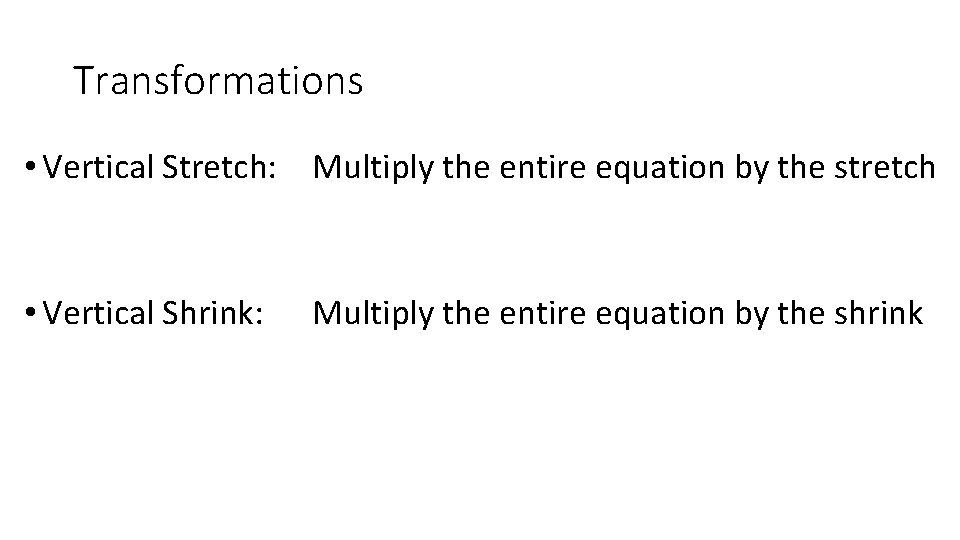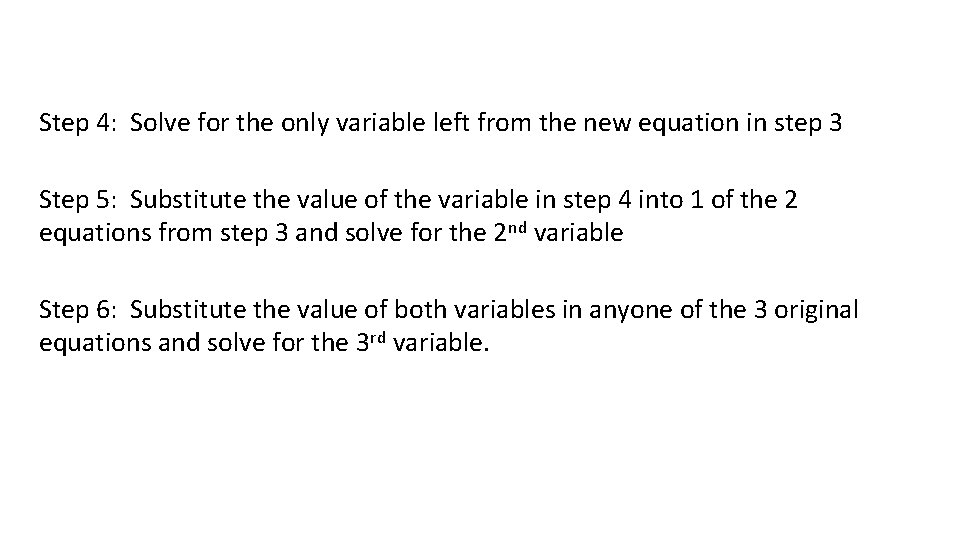# Formulas Slope Formula Slope Intercept Form Point Slope

• Slides: 7Formulas Slope Formula Slope Intercept Form Point Slope FormShifts • Shift right or left: Subtract or Add to just the X • Shift up or down: Add or Subtract to the whole equationTransformations • Horizontal Stretch: Multiply just the X • Example: Stretch by 2 then you multiply the X by 1/2 • Horizontal Shrink: Multiply just the X • Example: Shrink by 1/3 then you multiply the X by 3Transformations • Vertical Stretch: Multiply the entire equation by the stretch • Vertical Shrink: Multiply the entire equation by the shrinkReflections • Reflect over the X-axis • Multiply the entire equation by negative 1 • Reflect over the Y-axis • Multiply just the X by negative 1Steps to solving systems Step 1: Take 2 of the equations and get 1 variable to cancel out Step 2: Take the equation not used, and 1 of the equations just used, and get the same variable to cancel out Step 3: Take the 2 new equations from steps 1 and 2 (which should have only 2 variables in each), and get 1 of those variables to cancel outStep 4: Solve for the only variable left from the new equation in step 3 Step 5: Substitute the value of the variable in step 4 into 1 of the 2 equations from step 3 and solve for the 2 nd variable Step 6: Substitute the value of both variables in anyone of the 3 original equations and solve for the 3 rd variable.